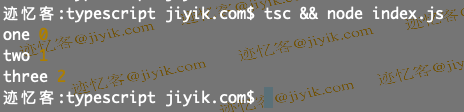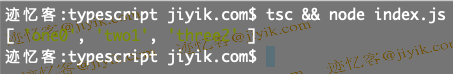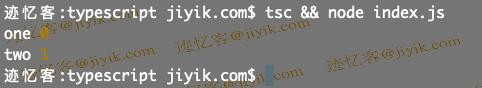# 迹忆客专注技术分享

## 在 TypeScript 中使用索引遍历数组

``````const arr: string[] = ['one', 'two', 'three'];

arr.forEach((element, index) => {
// 👇️ one 0, two 1, three 2
console.log(element, index);
});
``````1. 数组中的当前元素。
2. 数组中元素的索引。
3. 我们调用 `forEach()` 方法的数组。

`forEach` 方法返回 undefined，因此它用于改变外部变量。

``````const arr: string[] = ['one', 'two', 'three'];

const result = arr.map((element, index) => {
return element + index;
});

// 👇️ ['one0', 'two1', 'three2']
console.log(result);
```````map()` 方法返回一个新数组，其中包含我们从回调函数返回的元素。

``````const arr: string[] = ['one', 'two', 'three'];

for (let index = 0; index < arr.length; index++) {
// 👇️ one 0, two 1
console.log(arr[index], index);

if (index === 1) {
break;
}
}
``````## 相关文章

### 在 TypeScript 中把 JSON 对象转换为一个类## 热门标签